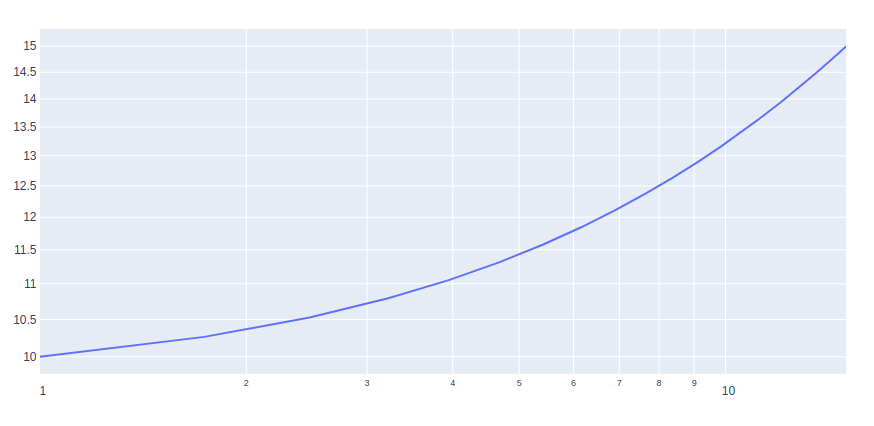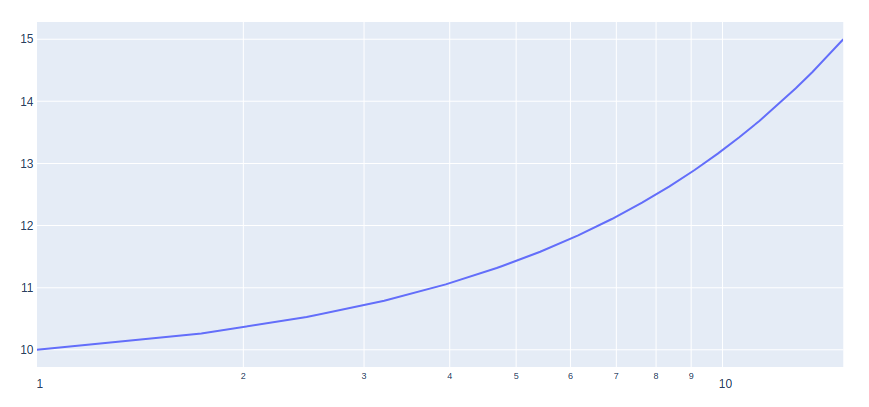Open in App
Not now

# How to make Log Plots in Plotly – Python?

• Last Updated : 05 Sep, 2020

A Plotly is a Python library that is used to design graphs, especially interactive graphs. It can plot various graphs and charts like histogram, barplot, boxplot, spreadplot, and many more. It is mainly used in data analysis as well as financial analysis. plotly is an interactive visualization library.

## What are the log plots?

A log plot is a way of displaying numerical data over a very wide range of values in a compact way. There are two types of plots:

• log-log plot: If both the vertical and horizontal axes of a plot are scaled logarithmically, the plot is referred to as a log-log plot.
• Semi-logarithmic plots: If only the ordinate or abscissa is scaled logarithmically, the plot is referred to as a semi-logarithmic plot.

### Creating Log plots using plotly

There is no specific function provided for creating the log plots. However, it can be created using the scatter() method of graph_objects class. We will have to change the xaxis_type and yaxis_type to log.

Example 1: log-log plot

## Python3

 `import` `plotly.graph_objects as go``import` `numpy as np`` ` ` ` `x ``=` `np.linspace(``1``, ``15``, ``20``)``y ``=` `np.linspace(``10``, ``15``, ``20``)`` ` `fig ``=` `go.Figure()`` ` `fig.add_trace(go.Scatter(``    ``x``=``x,``    ``y``=``y``))`` ` `fig.update_layout(xaxis_type``=``"log"``, yaxis_type``=``"log"``)``fig.show()`

Output:Example 2: semi-log plot

## Python3

 `import` `plotly.graph_objects as go``import` `numpy as np`` ` ` ` `x ``=` `np.linspace(``1``, ``15``, ``20``)``y ``=` `np.linspace(``10``, ``15``, ``20``)`` ` `fig ``=` `go.Figure()`` ` `fig.add_trace(go.Scatter(``    ``x``=``x,``    ``y``=``y``))`` ` `fig.update_layout(xaxis_type``=``"log"``)``fig.show()`

Output:My Personal Notes arrow_drop_up